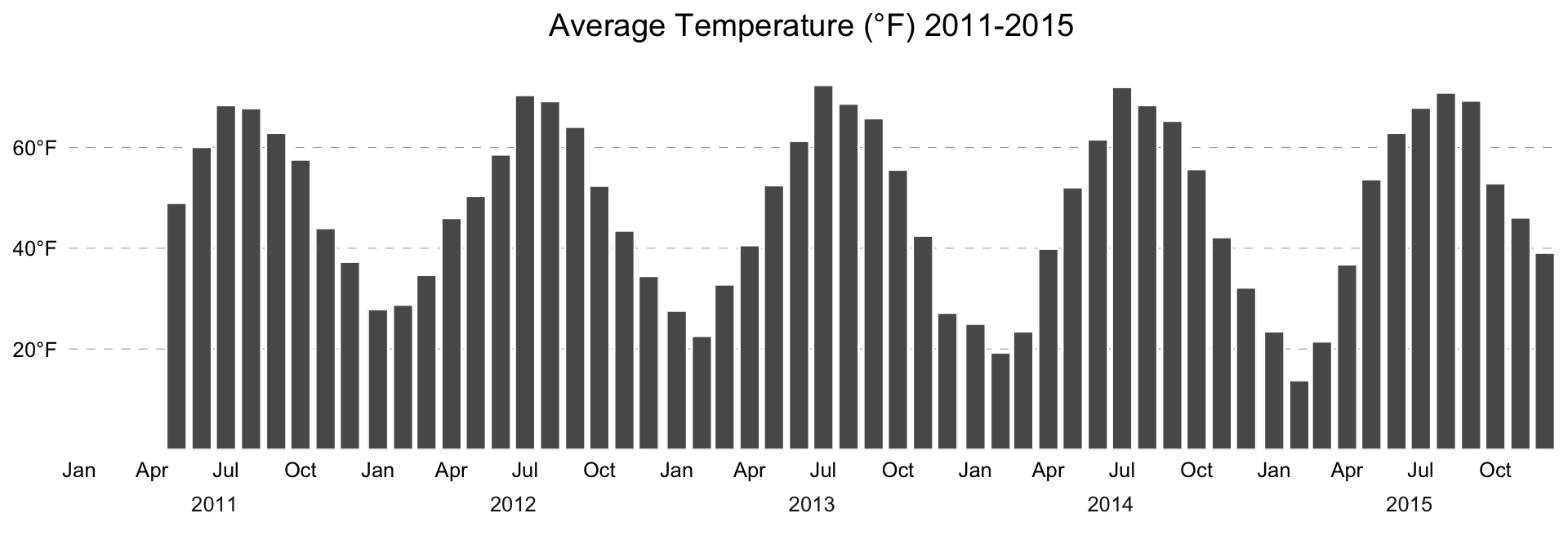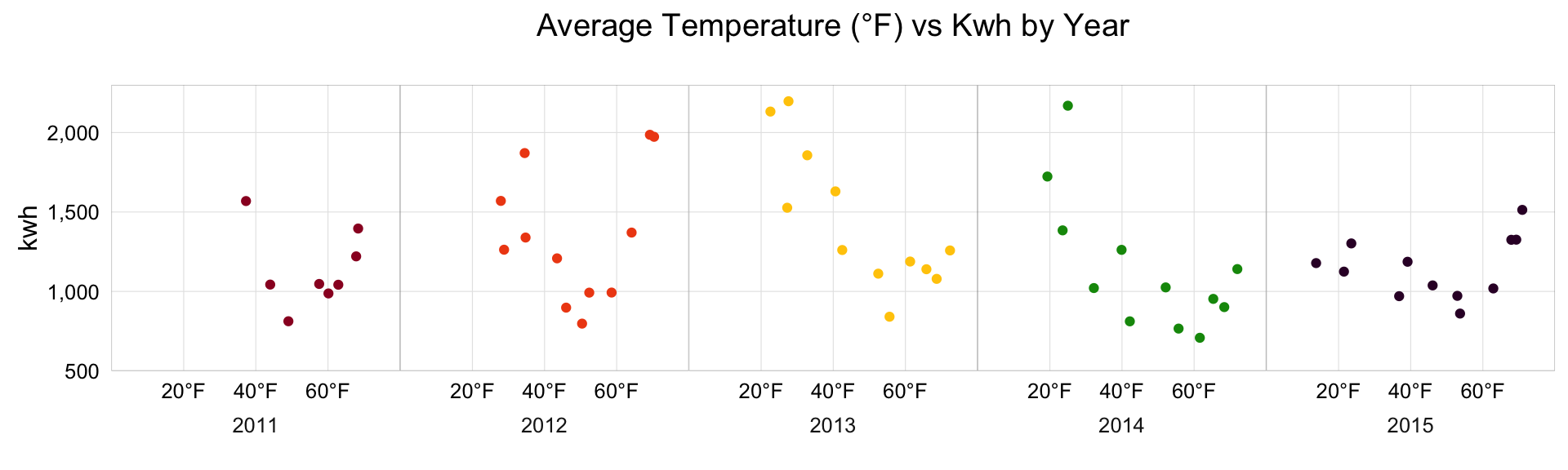ggplot2 2.0 provides new facet labeling options that make it possible to create beautiful small multiple plots or panel charts without resorting to icky grob manipulation.

require(jsonlite)
require(ggplot2)
require(dplyr)
require(scales)
require(ggthemes)
require(colourlovers)
require(grid)

My power company uses a third party service which enables me to see our power usage on a portal. Thankfully, that portal uses javascript charts and retreives the data via an XHR request that returns usable JSON.

power <- fromJSON("power.json")
pwr_samp <- power\$samples[]
pwr_samp <- mutate(pwr_samp,
date=as.Date(date, "%Y-%m-%d"),
yr=format(date, "%Y"))

My data doesn’t go back before May, so I need to fill in that gap:

bind_rows(pwr_samp,
data.frame(date=seq(as.Date("2011-01-01"),
as.Date("2011-04-01"),
"1 month"),
yr="2011", kwh=0, temp_avg_f=0,
stringsAsFactors=FALSE)) -> pwr_samp

And, there’s a dup for 2011-11-01, so I also need to handle that:

pwr_samp <- distinct(pwr_samp, date)

I also want prettier Y-axis labels:

degf <- function(x, ...) { paste(x, "°F", sep="") }

A horrible stacked bar chart posted on Twitter last week made me want to use a “skittles” palette

skittles <- swatch(clpalette(1521357))[]

First, I’ll make a bar chart of avg monthly temps over time. By fine-tuning the breaks and performing some minor adjustments with the facet options I can get a nice bar chart that looks like it is one solid chart but in reality is faceted by year

gg <- ggplot(pwr_samp)
gg <- gg + geom_hline(yintercept=c(20, 40, 60), size=0.15, linetype="dashed", alpha=1/2)
gg <- gg + geom_bar(stat="identity", aes(x=date, y=temp_avg_f), color="white")
gg <- gg + scale_x_date(expand=c(0,0))
gg <- gg + scale_y_continuous(expand=c(0,0), breaks=c(20, 40, 60), label=degf)
gg <- gg + facet_wrap(~yr, switch="x", ncol=5, scales="free_x")
gg <- gg + labs(x=NULL, y=NULL, title="Average Temperature (°F) 2011-2015\n")
gg <- gg + theme_bw()
gg <- gg + theme(axis.ticks.y=element_blank())
gg <- gg + theme(axis.ticks.x=element_blank())
gg <- gg + theme(panel.grid=element_blank())
gg <- gg + theme(panel.margin.x=unit(0.125, "cm"))
gg <- gg + theme(strip.background=element_blank())
gg <- gg + theme(panel.border=element_blank())
ggIt’s also possible to keep the appearance of individual panels, but make them a bit more visually appealing (both the panels and the axis labels)

gg <- ggplot(pwr_samp)
gg <- gg + geom_jitter(aes(x=temp_avg_f, y=kwh, color=yr))
gg <- gg + facet_wrap(~yr, switch="x", ncol=5)
gg <- gg + labs(x=NULL, title="Average Temperature (°F) vs Kwh by Year\n")
gg <- gg + scale_x_continuous(expand=c(0,0), label=degf,
breaks=c(20, 40, 60), limits=c(-0.05, 80))
gg <- gg + scale_y_continuous(expand=c(0,0), label=comma, limits=c(500, 2300))
gg <- gg + scale_color_manual(values=skittles)
gg <- gg + theme_bw()
gg <- gg + theme(strip.background=element_blank())
gg <- gg + theme(panel.grid.minor=element_blank())
gg <- gg + theme(axis.ticks=element_blank())
gg <- gg + theme(panel.margin=unit(0, "null"))
gg <- gg + theme(panel.border=element_rect(color="#7f7f7f88", size=0.25))
gg <- gg + theme(legend.position="none")
gg